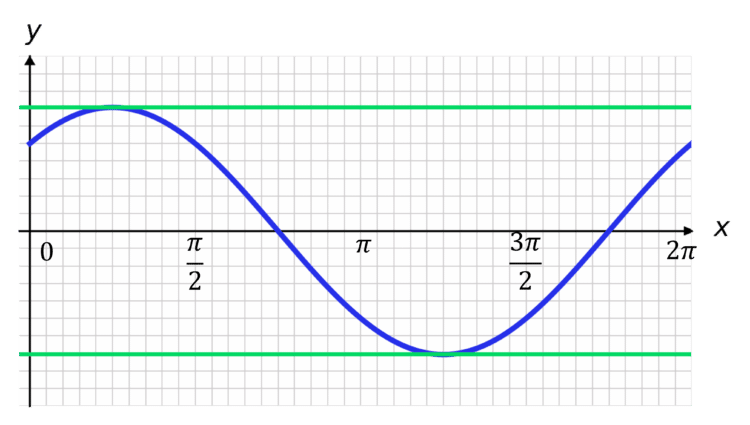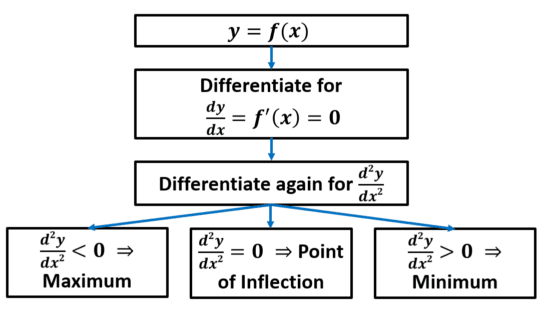# Stationary Points

A LevelAQAEdexcelOCR

## Stationary Points

When $\dfrac{df(x)}{dx}>0$, the function $f(x)$ is increasing.

When $\dfrac{df(x)}{dx}<0$, the function $f(x)$ is decreasing.

A stationary point of a function is when it is neither increasing – i.e. when $\dfrac{df(x)}{dx}=0$

Make sure you are happy with the following topics before continuing.

A LevelAQAEdexcelOCR

## Finding Stationary Points

To find a point where the gradient is $0$, we need to set $\dfrac{dy}{dx} = f'(x) = 0$.

So, let’s say we’ve got a nice, easy function: $\textcolor{blue}{y = x^2 - 1}$.Well, we know that the gradient is given by $\dfrac{dy}{dx} = 2x$.

Setting $\textcolor{limegreen}{\dfrac{dy}{dx} = 2x = 0}$ gives $x = 0$, for which $y = 0^2 - 1 = -1$.

We conclude that $\textcolor{blue}{y = x^2 - 1}$ has a stationary point at $(0, -1)$.

A LevelAQAEdexcelOCR

## Finding Stationary Points of Complicated Functions

Let $\textcolor{blue}{y = \sin x + \cos x}$ for $x$ measured in radians. How might we go about finding the stationary points (in the range
$0 \leq x \leq 2\pi$)?$\textcolor{blue}{y = \sin x + \cos x}$ gives $\dfrac{dy}{dx} = \cos x - \sin x$.

Setting $\textcolor{limegreen}{\dfrac{dy}{dx} = 0}$, we require $\cos x = \sin x$, or, $\tan x = 1$.

This gives $x = \tan ^{-1}(1) = \dfrac{\pi}{4}, \dfrac{5\pi}{4}$.

Therefore, for $0 \leq x \leq 2\pi$, we have the stationary points $\left( \dfrac{\pi}{4}, \sqrt{2}\right) , \left( \dfrac{5\pi}{4}, -\sqrt{2}\right)$.

A LevelAQAEdexcelOCR

## Finding the Nature of Stationary Points

Once we’ve found our stationary points, we need to find out whether they are a maximum, minimum, or a stationary point of inflection.

We do this by differentiating our derivative again.So, for example, take our first example of $y = x^2 - 1$.

We said that $\dfrac{dy}{dx} = 2x$.

So $\dfrac{d^{2}y}{dx^2} = 2$.

Since $\dfrac{d^{2}y}{dx^2} = 2 > 0$, we can conclude that this is a minimum point. You probably figured that out from the graph, though.

What about the second example, $y = \sin x + \cos x$?

Well, we know that $\dfrac{dy}{dx} = \cos x - \sin x$.

By extension, $\dfrac{d^{2}y}{dx^2} = -\sin x - \cos x$. We now need to test both of our values of $x$ for the corresponding value of $\dfrac{d^{2}y}{dx^2}$.

So, for $x = \dfrac{\pi}{4}$, $\dfrac{d^{2}y}{dx^2} = -\sqrt{2} < 0$, so it is a maximum point.

For $x = \dfrac{5\pi}{4}$, $\dfrac{d^{2}y}{dx^2} = \sqrt{2} > 0$, so it is a minimum point.

A LevelAQAEdexcelOCR

## Note

$\dfrac{d^{2}y}{dx^{2}}=0$ doesn’t always mean the stationary point is a point of inflection.

For example, $y=x^{4}$ has a minimum at $x=0$, but $\dfrac{d^{2}y}{dx^{2}}=12x^{2}$, which equals $0$ at $x=0$, so in this case $\dfrac{d^{2}y}{dx^{2}}=0$ leads to a minimum.

However, all points of inflection have the property $\dfrac{d^{2}y}{dx^{2}}=0$, so to interpret the result $\dfrac{d^{2}y}{dx^{2}}=0$ you will need to check whether the second derivative changes sign either side of the stationary point.

## Sketching Graphs from Information about Functions

Say we have a complex function with multiple terms, i.e. $\textcolor{blue}{f(x) = 1 + x - 2\cos x}$ where $-2\pi \leq x \leq 2\pi$ is measured in radians.

Then $f'(x) = 1 + 2\sin x$ and $f''(x) = 2\cos x$.

Therefore, any stationary points will occur when $\sin x = \dfrac{-1}{2}$, so $x = \dfrac{-5\pi}{6}, \dfrac{-\pi}{6}, \dfrac{7\pi}{6}, \dfrac{11\pi}{6}$.

For $x = \dfrac{-5\pi}{6}$, $f(x) = 0.114$ and $f''(x) = -\sqrt{3}$, which is a maximum.

For $x = \dfrac{-\pi}{6}$, $f(x) = -1.256$ and $f''(x) = \sqrt{3}$, which is a minimum.

For $x = \dfrac{7\pi}{6}$, $f(x) = 6.397$ and $f''(x) = -\sqrt{3}$, which is a maximum.

For $x = \dfrac{11\pi}{6}$, $f(x) = 5.028$ and $f''(x) = \sqrt{3}$, which is a minimum.

We can sketch these parts onto a graph to give us a rough form.We can be helped further in sketching by considering the behaviour of the graph as $x$ gets really big or really small. We know that the graph roughly follows $y=x+1$, so as $x$ gets really big so does $y$, and as $x$ gets really small so does $y$. With this, we can finish our graph.A LevelAQAEdexcelOCR

## Stationary Points Example Questions

$f(x) = x^2 + 3x - 4$

gives

$f'(x) = 2x + 3$

$f'(x) = 0$ when $x = -\dfrac{3}{2}$.

Substituting this value into $f(x)$ gives $y=-\dfrac{25}{2}$

Thus, the stationary point is at $\bigg(-\dfrac{3}{2}, -\dfrac{25}{4}\bigg)$

$y = 9 - x^2$ gives

$\dfrac{dy}{dx} = -2x$

$\dfrac{d^2y}{dx^2} = -2$

$-2 < 0$, so this is a maximum point.

$f(x) = x^4 + \dfrac{8}{3}x^3 - 10x^2 - 24x$

$f'(x) = 4x^3 + 8x^2 - 20x - 24$

$f'(x) = 4x^3 + 8x^2 - 20x - 24 = 0$ can be simplified to $x^3 + 2x^2 - 5x - 6 = 0$

$x^3 + 2x^2 - 5x - 6 = (x + 1)(x^2 + x - 6) = (x + 1)(x - 2)(x + 3) = 0$

So, $f'(x) = 0$ has solutions at $x = -3, -1, 2$.

$f''(x) = 12x^2 + 16x - 20$

So

$f''(x) = 40 > 0$ when $x = -3$, so this is a minimum point.

$f''(x) = -24 < 0$ when $x = -1$, so this is a maximum point.

$f''(x) = 60 > 0$ when $x = 2$, so this is a minimum point.

A Level

A Level

A Level

A Level

## You May Also Like...### MME Learning Portal

Online exams, practice questions and revision videos for every GCSE level 9-1 topic! No fees, no trial period, just totally free access to the UK’s best GCSE maths revision platform.

£0.00

A Level

A Level